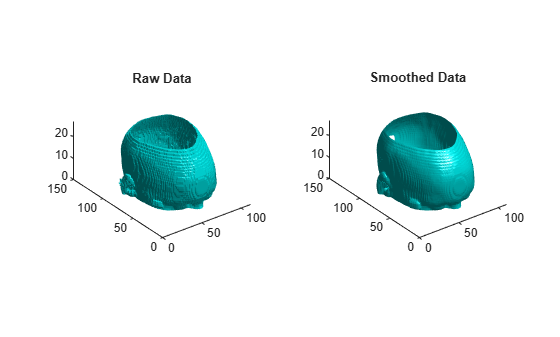# smooth3

Smooth 3-D data

## Syntax

``W = smooth3(V)``
``W = smooth3(V,method)``
``W = smooth3(V,method,size)``
``W = smooth3(V,method,size,sd)``

## Description

example

````W = smooth3(V)` smooths the volumetric data `V` and returns the smoothed data in `W`. `W` is a double array with the same dimensions as `V`. ```
````W = smooth3(V,method)` uses the specified smoothing method to define the convolution kernel and smooth the data.```

example

````W = smooth3(V,method,size)` specifies the 3-D window size for the smoothing method.```
````W = smooth3(V,method,size,sd)` specifies the standard deviation for the `"gaussian"` smoothing method.```

## Examples

collapse all

Load the `mri` data set and squeeze the 4-D array stored in the `D` variable into three dimensions. Then smooth the data.

```load mri D = squeeze(D); W = smooth3(D);```

Display the raw data and the smoothed data as an isosurface.

```figure tiledlayout(1,2) nexttile p1 = patch(isosurface(D,5),"FaceColor","cyan", ... "EdgeColor","none"); view(3) daspect([1,1,0.4]) camlight isonormals(D,p1) title("Raw Data") nexttile p2 = patch(isosurface(W,5),"FaceColor","cyan", ... "EdgeColor","none"); view(3) daspect([1,1,0.4]) camlight isonormals(W,p2) title("Smoothed Data")```Create a 10-by-10-by-10 array of random data. Smooth the data using the `"gaussian"` method with a 3-D window size of 5.

```data = rand(10,10,10); data = smooth3(data,"gaussian",5);```

Display the data as an isosurface with end caps.

```patch(isocaps(data,0.5), ... "FaceColor","interp","EdgeColor","none") p1 = patch(isosurface(data,0.5), ... "FaceColor","blue","EdgeColor","none"); isonormals(data,p1) view(3) axis vis3d tight camlight left lighting gouraud```## Input Arguments

collapse all

Volumetric data, specified as a 3-D array.

Data Types: `single` | `double` | `int8` | `int16` | `int32` | `int64` | `uint8` | `uint16` | `uint32` | `uint64` | `logical`

Smoothing method, specified as one of these filters:

• `"box"` — Weighted moving average over each window of `V`

• `"gaussian"` — Gaussian-weighted moving average over each window of `V`

The smoothing method determines the convolution kernel.

Window size of the selected smoothing method, specified as a three-element vector of positive odd integers or a positive off integer scalar. If `size` is scalar, then `size` is interpreted as ```[size size size]```.

The window size determines how much smoothing is applied to the data. As the window size increases, more data points are used for the averaging process, and therefore more smoothing occurs.

When using the `"gaussian"` smoothing method, both standard deviation and window size determine how much smoothing is applied to the data.

Standard deviation for the `"gaussian"` smoothing method, specified as a numeric value. As the standard deviation value increases, more averaging is applied within the filter window.

If the smoothing method is set to `"box"`, `sd` has no effect.

Data Types: `single` | `double`

## Version History

Introduced before R2006a